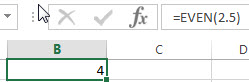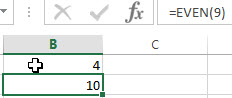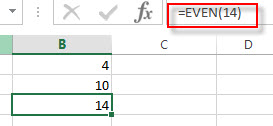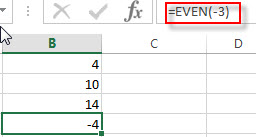## Excel EVEN function

This post will guide you how to use Excel EVEN function with syntax and examples in Microsoft excel.

### Description

The Excel EVEN function rounds a given number up to the nearest even integer. So you can use the EVEN function to return the next even integer after rounding a supplied number up. The returned value is away from zero. It means that if the number is a positive value and the return value will become larger and if the number is a negative value, the return value will become smaller.

The EVEN function is a build-in function in Microsoft Excel and it is categorized as a Math and Trigonometry Function.

The EVEN function in Excel 2016, Excel 2013, Excel 2010, Excel 2007, Excel 2003, Excel XP, Excel 2000, Excel 2011 for Mac.

### Syntax

The syntax of the EVEN function is as below:

`=EVEN (number)`

Where the EVEN function arguments is:

• number -This is a required argument. . A numeric value that you want to round up to the nearest even integer.

Note:

• If the number argument is not numeric value, the EVEN function will return the #VALUE! Error.

### Excel EVEN Function Examples

The below examples will show you how to use Excel EVEN Function to round up a given number to the nearest even integer.

1# to round 2.5 up to the nearest even integer, enter the following formula in Cell B1.

`=EVEN(2.5)`2# to round 9 to the nearest even integer, enter the following formula in Cell B2.

`=EVEN(9)`3# to round 14 up to the nearest even integer, enter the following formula in Cell B3.

`=EVEN(14)`4# to round -3 up to the nearest even integer, enter the following formula in Cell B4.

`=EVEN(-3)`### Related Functions

• Excel ODD function
The Excel ODD function rounds a given number up to the nearest odd integer. So you can use the ODD function to return the next ODD integer after rounding a supplied number up. The syntax of the ODD function is as below:=ODD (number)…
• Excel ROUND function
The Excel ROUND function rounds a number to a specified number of digits. You can use the ROUND function to round to the left or right of the decimal point in Excel.The syntax of the ROUND function is as below:=ROUND (number, num_digits)…
• Excel ROUNDDOWN function
The Excel ROUNDDOWN function round the number down to the specified number of digits. The syntax of the ROUNDDOWN function is as below:=ROUNDDOWN (number, num_digits)…
• Excel ROUNDUP function
The Excel ROUNDUP function rounds the number up to a specified number of decimal places. It will round away from 0.The syntax of the ROUNDUP function is as below:=ROUNDUP (number, num_digits)…
• Excel CEILING Function
The Excel CEILING function returns a given number rounded up to the nearest multiple of a given number of significance. The syntax of the CEILING function is as below:= CEILING (number, significance)…
• Excel CEILING.PRECISE Function
The Excel CEILING.PRECISE function returns a given number rounded up to the nearest multiple of a given number of significance or returns a number that is rounded up to the nearest integer number.The syntax of the CEILING.PRECISE function is as below:=CEILING.PRECISE (number, [significance])…